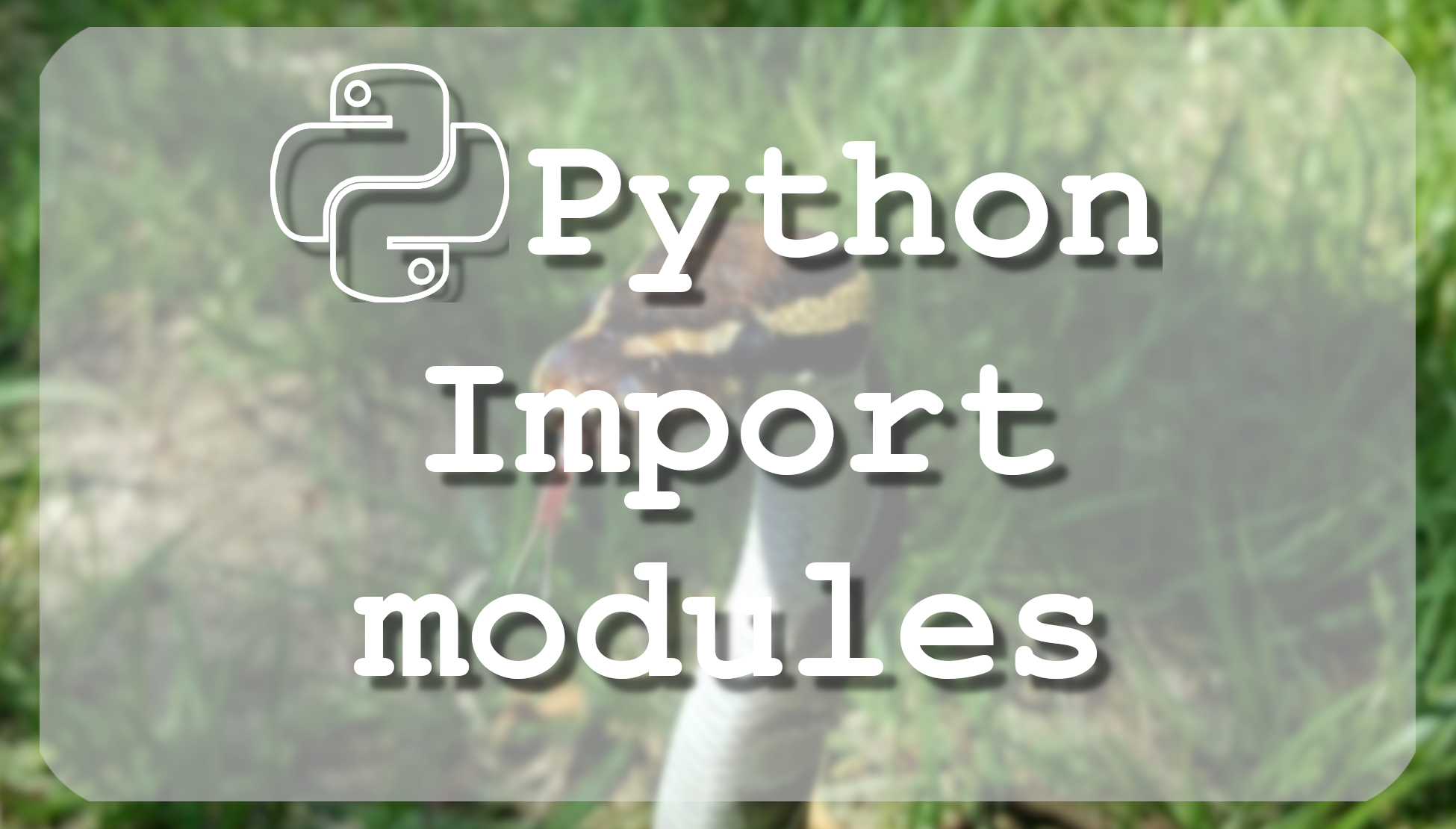# Tutorial - Python 3 basics Import Modules

## This tutorial is about Python variables, you will learn how to use global and local variables. This helps to keep code clean and easy to read

The Most Advanced Data Science Roadmaps You've Ever Seen! Comes with Thousands of Free Learning Resources and ChatGPT Integration! https://aigents.co/learn/roadmaps/intro

Python import module is an object with arbitrarily named attributes that you can bind and reference. Simply, a module is a file consisting of a Python code. A module can define functions, classes, and variables which include runnable code. If you are working on a large project, modules can help keep code clean and easy to read. So first, when you are importing a module to your code, you are basically loading that module into memory.

## Basic import syntax:

``import module1[, module2[,... moduleN]``

You can use any Python source file as a module by executing an import statement in some other Python source file. When the Python interpreter encounters an import statement, it imports the module if the module is present in the search path. A search path is a list of directories that the interpreter searches before importing a module.

When you import a module, the Python interpreter searches for the module in the following sequences:

• The current directory.
• If the module isn't found in the current directory, Python searches each directory in the shell variable PYTHONPATH.
• If the current directory and PYTHONPATH fail, Python checks the default path. On UNIX, this default path is normally `/usr/local/lib/python/`.

For example, to import the built-in module `statistics.py`, you need to put the following command at the top of the script:

``````# Import module statistics
import statistics``````

For example, we are creating a `printing.py` file with the following code:

``````def print_func(parameter):
print ("Printing:", parameter)
return``````

Then we can import our created module to our code:

``````# Import module printing
import printing

# Now you can call defined function that module as follows
printing.print_func("Welcome to import tutorial")``````

When the above code is executed, it will produce the following result: "`Printing: Welcome to import tutorial`".

Sometimes, you will see people use the "as" statement in their imports. This will allow you to rename the imported module to whatever you want. People generally do this to shorten the name of the module. Below is the same code example that was used above but here while importing we are using "as" and receiving the same result as before:

``````# Import module support
import printing as p

# Now you can call defined function that module as follows
p.print_func("Welcome to import tutorial")``````

## from...import statement:

Sometimes you don't want to import all modules to your python code, to avoid messy code, for example:

``````# Import module statistics
import statistics

example_list = [5,2,5,6,1,2,6,7,2,6,3,5,5]
print(statistics.variance(example_list))``````

Above is the messy code, and every time you want to use variance, you'll need to write `statistics.variance(...)`, in this case, it's better to import only the variance module:

``````from statistics import variance as var

example_list = [5,2,5,6,1,2,6,7,2,6,3,5,5]
print(var(example_list))``````

This statement does not import the entire statistics module into the current namespace. It just introduces the item variance from the module statistics into the global symbol table of the importing module and it's much easier to use it like that.

What if we want to import everything from statistics like we did initially, but we don't want to type the statistics because you don't want it or you don't have time for that, simple, we use ' * '. This way you will need to use the full module name, but this way you can learn these modules better.

``````from statistics import *

example_list = [5,2,5,6,1,2,6,7,2,6,3,5,5]
print(variance(example_list))``````

We must mention that when the module is imported into your python script, the code in the top-level portion of a module is executed only once. So if you are importing a file, where parameters are changing in time, your imported file will use only these parameters which were on importing time. In this case, you need to upload your code again.

So, if you want to execute the top-level code in a module, you can use reload() function. This function imports a previously imported module again. The syntax of the reload() function is this:

``reload(module_name)``

Here, module_name is the name of the module you want to reload and not the string containing the module name. For example, to reload the statistics module in your code, use the following code:

``reload(statistics)``

## The dir() function

There is one good to know built-in dir() function, which returns a sorted list of strings containing the names defined by a module.

The list contains the names of all the modules, variables and functions that are defined in a module. Here is a simple example:

``````# Import built-in statistics module
import statistics

content = dir(statistics)
print(content)``````

When the above code is executed, we'll get such result:

``````['Decimal', 'Fraction', 'StatisticsError', '__all__', '__builtins__', '__cached__',
'__doc__', '__file__', '__loader__', '__name__', '__package__', '__spec__', '_coerce',
'_convert', '_counts', '_exact_ratio', '_fail_neg', '_find_lteq', '_find_rteq', '_isfinite',
'_ss', '_sum', 'bisect_left', 'bisect_right', 'chain', 'collections', 'decimal', 'groupby',
'harmonic_mean', 'math', 'mean', 'median', 'median_grouped', 'median_high', 'median_low',
'mode', 'numbers', 'pstdev', 'pvariance', 'stdev', 'variance']``````

Here, the special string variable __name__ is the module's name, and __file__ is the filename from which the module was loaded. Here are much more modules, but as we are learning basics we don't need to know them.

In this tutorial, we covered how to use the import python module and what purpose has `reload()` and dir() functions.